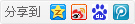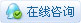##帐号 自动登录 找回密码 密码 立即注册→加入我们
 搜索 热搜: 下载 VB C 实现 编写

# 【VFB】程序（VFB教程2-7）

51主题 642积分

UID
3260

7

12 点

502 个

1 次

0 份

22 小时

2017-12-26发表于 2018-2-26 22:16:15 | 显示全部楼层 |阅读模式

### 欢迎访问技术宅的结界，请注册或者登录吧。

x

Sub
Sub 是不返回值的过程。它们使用Declare关键字声明，并使用Sub关键字定义。声明过程引入其名称，以便可以调用它，并且过程定义列出了在调用时将执行的代码语句。A sub 简单地通过在程序中的某个地方使用它的名称。
[Visual Basic] 纯文本查看 复制代码
Sub MyProcedure
Print "the body of MyProcedure"
End Sub

the body of MyProcedure

Functions
Functions 是将值返回到调用代码中的点的过程。您可以将function 调用视为对某个表达式的评估，就像变量或对象一样。它们使用Declare关键字声明，并使用Function关键字定义。在声明结尾处指定functions 返回的值的类型。
[Visual Basic] 纯文本查看 复制代码
' introduces and defines a procedure that returns an integer value
Function MyProcedure As Integer
Return 10
End Function

' calls the procedure, and stores its return value in a variable
Dim i As Integer = MyProcedure
Print i

10

[Visual Basic] 纯文本查看 复制代码
Sub Procedure (s As String, n As Integer)
Print "The parameters have the values: " & s & " and " & n
End Sub

Procedure "abc", 123

The parameters have the values: abc and 123

[Visual Basic] 纯文本查看 复制代码
Sub Procedure (ByVal param As Integer)
param *= 2
Print "The parameter 'param' = " & param
End Sub

Dim arg As Integer = 10
Print "The variable 'arg' before the call = " & arg
Procedure(arg)
Print "The variable 'arg' after the call = " & arg

The variable 'arg' before the call = 10
The parameter 'param' = 20
The variable 'arg' after the call = 10

[Visual Basic] 纯文本查看 复制代码
Sub Procedure (ByRef param As Integer)
param *= 2
Print "The parameter 'param' = " & param
End Sub

Dim arg As Integer = 10
Print "The variable 'arg' before the call = " & arg
Procedure(arg)
Print "The variable 'arg' after the call = " & arg

The variable 'arg' before the call = 10
The parameter 'param' = 20
The variable 'arg' after the call = 20

[Visual Basic] 纯文本查看 复制代码
Sub f( ByRef i As Integer )
i = 456
End Sub

Dim i As Integer = 123
Dim pi As Integer Ptr = @i

Print i
f( ByVal pi )
Print i

...指的是Function过程在函数完成时具有值，使得该值可以在表达式中使用或赋值给变量的能力。

[Visual Basic] 纯文本查看 复制代码
'' Using the name of the function to set the return value and continue executing the function:
Function myfunc1() As Integer
myfunc1 = 1
End Function

'' Using the keyword 'Function' to set the return value and continue executing the function:
Function myfunc2() As Integer
Function = 2
End Function

'' Using the keyword 'Return' to set the return value and immediately exit the function:
Function myfunc3() As Integer
Return 3
End Function
'' This program demonstrates a function returning a value.

Declare Function myFunction () As Integer

Dim a As Integer

'Here we take what myFunction returns and add 10.
a = myFunction() + 10

'knowing that myFunction returns 10, we get 10+10=20 and will print 20.
Print a

Function myFunction () As Integer
'Here we tell myFunction to return 10.
Function = 10
End Function

[Visual Basic] 纯文本查看 复制代码
Dim Shared i As Integer = 123

Function f( ) ByRef As Integer
Dim pi As Integer Ptr = @i

Function = ByVal pi

'' or, with RETURN it would look like this:
Return ByVal pi
End Function

Print i, f( )本版积分规则 回帖并转播 回帖后跳转到最后一页|申请友链||Archiver|手机版|小黑屋|技术宅的结界 ( 滇ICP备16008837号 )|网站地图

GMT+8, 2020-9-26 05:59 , Processed in 0.094238 second(s), 32 queries , Gzip On.